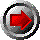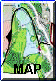The Woodland Education Centre - Woodland Restoration Project

METHODS

Each of the sampling areas consisted of a square with sides of 20m. This size was chosen because it was the largest square which would fit into the areas represented by Squares 3 and 4. A different shaped sampling unit could have been chosen to cover a larger area. This was not done because the shape of a sampling unit affects the results obtained. In most ecological surveys, the standard sampling shape is a square quadrat. It was therefore judged better to keep to a shape which would be readily comparable. The squares were all of the same size, because variations in the size of the sampling unit will also affect results.

The squares were laid out with corner posts connected by highly visible line to demarcate the area. Compass bearings were used to ensure that the lines were straight as they were laid. Squares 1 and 2 were laid out far enough away from the perimeter path (Fig. 2) to avoid the edge effect. (This edge effect results in local differences in vegetation cover due to the higher light levels on the path.)

Within each 20m square, the number of individual trees of different species was directly counted. Chalk was used to mark each tree as it was counted, to ensure that they were not missed, or counted twice. The girth of each tree was measured. (Tree girth is the circumference of the main trunk at breast height, which is taken to be a standard 1.5m from the ground.)

The height of trees in squares 3 and 4 was measured accurately using a clinometer (see Appendix A for details). Trees in squares 1 and 2 could not be measured using this method, because of the dense nature of the surrounding vegetation. Tree heights in these squares were therefore estimated. The accuracy of the estimates was checked by estimating the height of trees of known height from squares 3 and 4. Estimation was accurate to a level +/- 2m.

Other vegetation inside the squares was sampled within 2m2 quadrats. Ten quadrats were sampled in each of squares 1-4.

Ideally, the quadrats should have been randomly located within the 20m squares. However, in squares 1, 2 and 4 it proved impossible to place the quadrats randomly because of the difficulty of access. Instead, the quadrats were sited so that most areas of the 20m square were sampled. In square 3, where there was free access because the rhododendron had already been removed, the quadrats were randomly placed. This was accomplished using a numbered grid system and numbers selected from a random number table (see Appendix B for details). This method will be used in all squares in subsequent surveys, once the rhododendron has been cleared.

Within each quadrat sampled, the number of different rhododendron plants touching the quadrat were counted. Height of the rhododendron was estimated (accuracy +/- 1m). Girth was not measured because the rhododendron generally took the form of inumerable stems arising from a point and then horizontally interlinking with other neighbouring stems. There was thus rarely a single main stem and they could not be measured at a standard height.

Any other plants growing within the quadrat were counted and their heights measured. With the exception of square 3, in practice, there were rarely any other plants present, except occasionally a few mosses. Where these occurred, they were identified and the area covered by each species within the quadrat was measured. From this, the percentage of the quadrat area covered by the species was calculated.

The depth of the litter layer was also measured at several points within each quadrat and a mean value for litter depth within the quadrat calculated. The litter layer was measured down to the point at which leaf particles became so small as to be unrecognizable (corresponding to layers L and F in a soil profile). The proportion of leaf litter attributable to different plant species was estimated.

Comparative light levels in different regions of the project site were measured using a 35mm camera. A fixed aperture of 5.6 was set and shutter speed readings were taken at several different points within each of the four regions. A mean value for each region was then calculated. Shutter speed readings were also taken where there was bare sky and therefore full light penetration. All readings were taken at the same time and under the same conditions. The bare sky reading was taken as being 100% light penetration. The other regions were then compared to this level to obtain the percentage of light which managed to filter through the rhododendron canopy.

Results & DiscussionCONTENTS Introduction Site Description Methods Results & Discussion Conclusions Acknowledgements